Скачать презентацию Super-Resolution With Fuzzy Motion Estimation Matan Protter

0bb535a426969519d2ef0be9a43125eb.ppt

• Количество слайдов: 25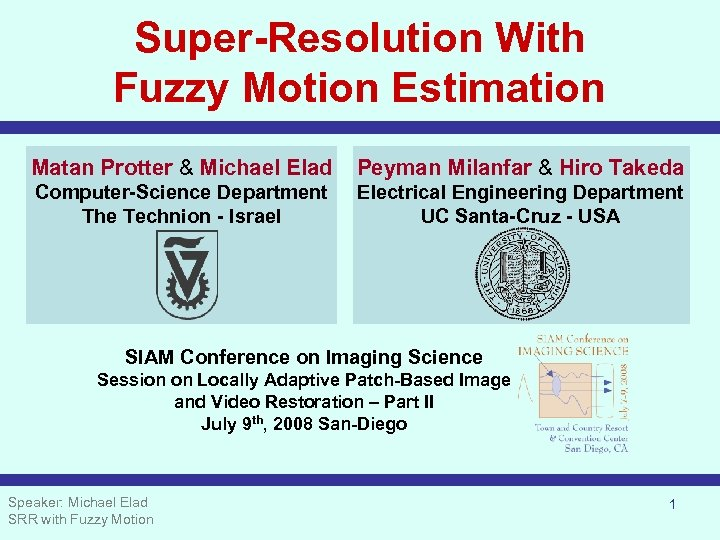Super-Resolution With Fuzzy Motion Estimation Matan Protter & Michael Elad Peyman Milanfar & Hiro Takeda Computer-Science Department The Technion - Israel Electrical Engineering Department UC Santa-Cruz - USA SIAM Conference on Imaging Science Session on Locally Adaptive Patch-Based Image and Video Restoration – Part II July 9 th, 2008 San-Diego Speaker: Michael Elad SRR with Fuzzy Motion 1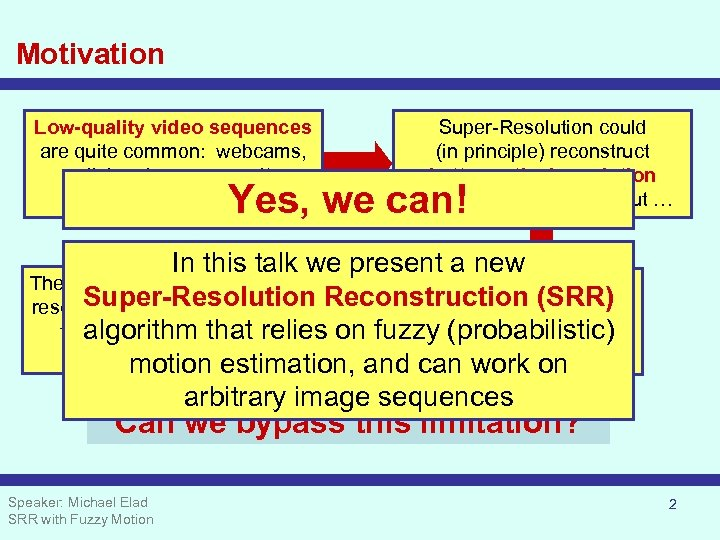Motivation Low-quality video sequences are quite common: webcams, cellular phones, security cameras, … Super-Resolution could (in principle) reconstruct better optical resolution from these sequences, but … Yes, we can! In this talk we present a new The implication: classical Super. This reconstruction Super-Resolution resolution algorithms are limited Reconstruction (SRR) requires highly to handle sequences with algorithm that relies on fuzzy (probabilistic) accurate motion global motion estimation, and can work on arbitrary image sequences Can we bypass this limitation? Speaker: Michael Elad SRR with Fuzzy Motion 2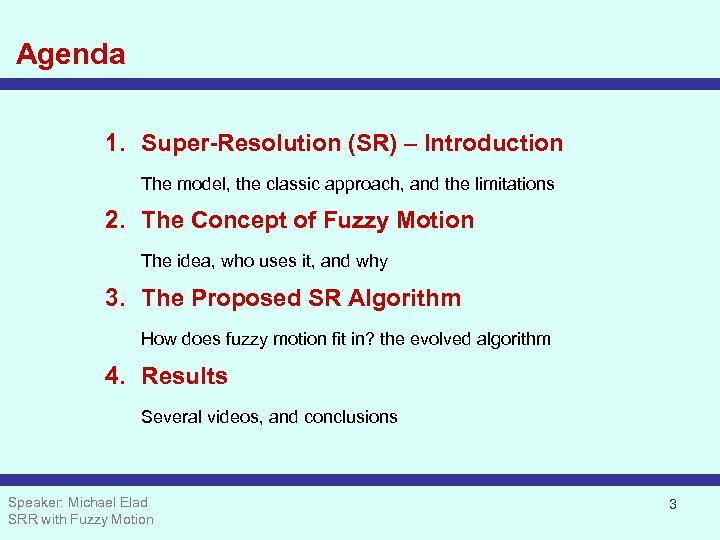Agenda 1. Super-Resolution (SR) – Introduction The model, the classic approach, and the limitations 2. The Concept of Fuzzy Motion The idea, who uses it, and why 3. The Proposed SR Algorithm How does fuzzy motion fit in? the evolved algorithm 4. Results Several videos, and conclusions Speaker: Michael Elad SRR with Fuzzy Motion 3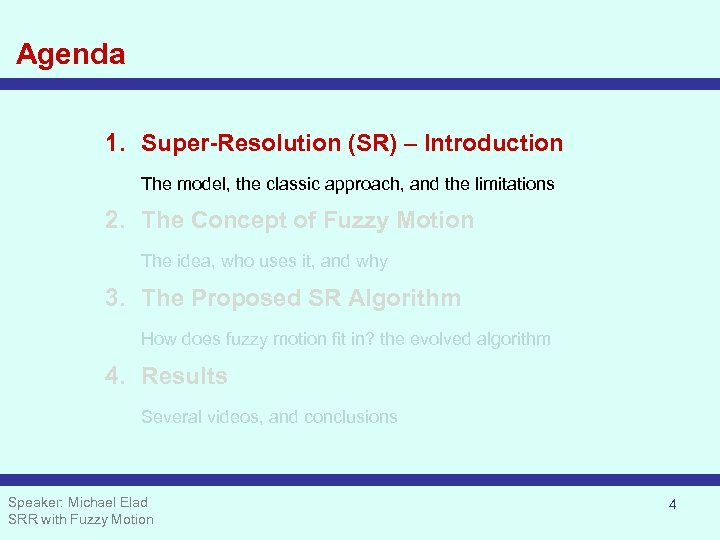Agenda 1. Super-Resolution (SR) – Introduction The model, the classic approach, and the limitations 2. The Concept of Fuzzy Motion The idea, who uses it, and why 3. The Proposed SR Algorithm How does fuzzy motion fit in? the evolved algorithm 4. Results Several videos, and conclusions Speaker: Michael Elad SRR with Fuzzy Motion 4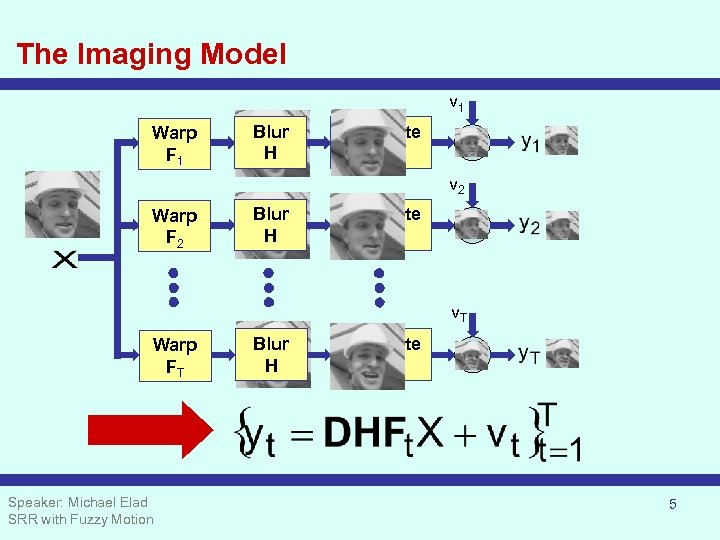The Imaging Model v 1 Warp F 1 Blur H Decimate D v 2 Warp F 2 Blur H Decimate D v. T Warp FT Speaker: Michael Elad SRR with Fuzzy Motion Blur H Decimate D 5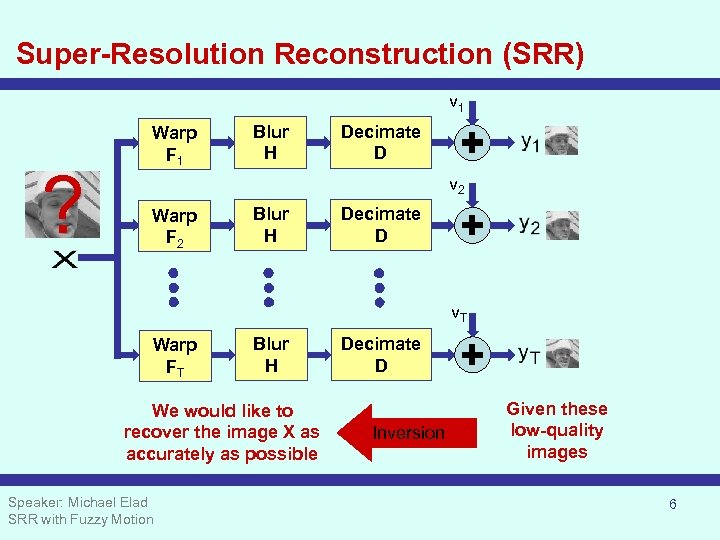Super-Resolution Reconstruction (SRR) v 1 ? Warp F 1 Blur H Decimate D v 2 Warp F 2 Blur H Decimate D v. T Warp FT Blur H We would like to recover the image X as accurately as possible Speaker: Michael Elad SRR with Fuzzy Motion Decimate D Inversion Given these low-quality images 6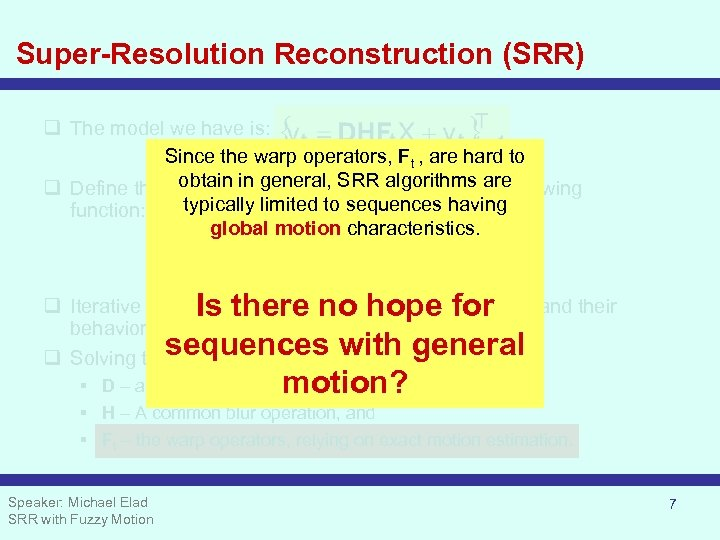Super-Resolution Reconstruction (SRR) q The model we have is: Since the warp operators, Ft , are hard to obtain in general, SRR algorithms are q Define the desired image as the minimizer of the following typically limited to sequences having function: global motion characteristics. q Iterative solvers can be applied for this minimization, and their Is there no hope for behavior is typically satisfactory, BUT … sequences knowledge of: q Solving the above requires thewith general motion? § D – a common decimation operation, § H – A common blur operation, and § Ft – the warp operators, relying on exact motion estimation. Speaker: Michael Elad SRR with Fuzzy Motion 7SRR – Just a Small Example 3: 1 scale-up in each axis using 9 images, with pure global translation between them Speaker: Michael Elad SRR with Fuzzy Motion 8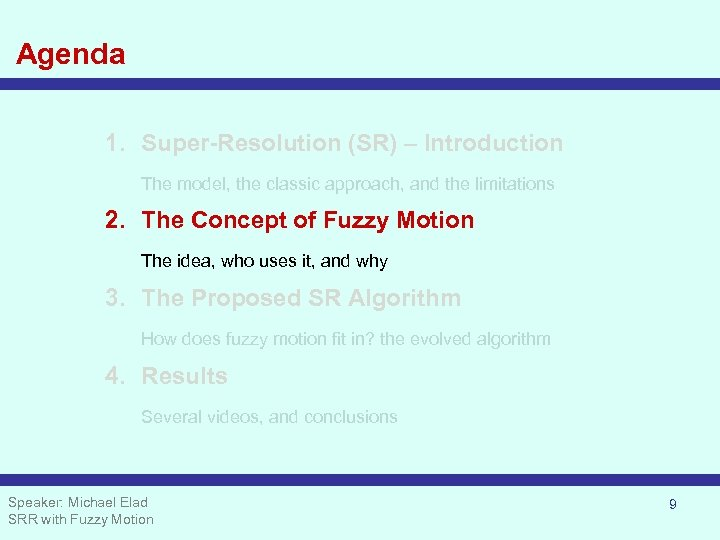Agenda 1. Super-Resolution (SR) – Introduction The model, the classic approach, and the limitations 2. The Concept of Fuzzy Motion The idea, who uses it, and why 3. The Proposed SR Algorithm How does fuzzy motion fit in? the evolved algorithm 4. Results Several videos, and conclusions Speaker: Michael Elad SRR with Fuzzy Motion 9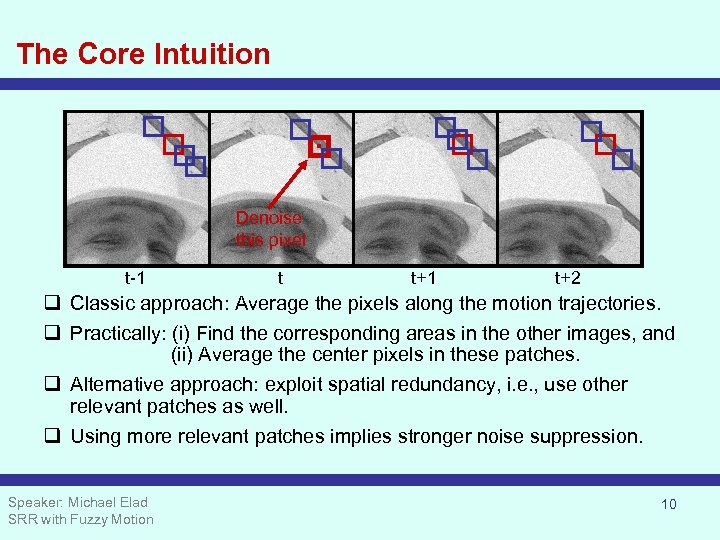The Core Intuition Denoise this pixel t-1 t t+1 t+2 q Classic approach: Average the pixels along the motion trajectories. q Practically: (i) Find the corresponding areas in the other images, and (ii) Average the center pixels in these patches. q Alternative approach: exploit spatial redundancy, i. e. , use other relevant patches as well. q Using more relevant patches implies stronger noise suppression. Speaker: Michael Elad SRR with Fuzzy Motion 10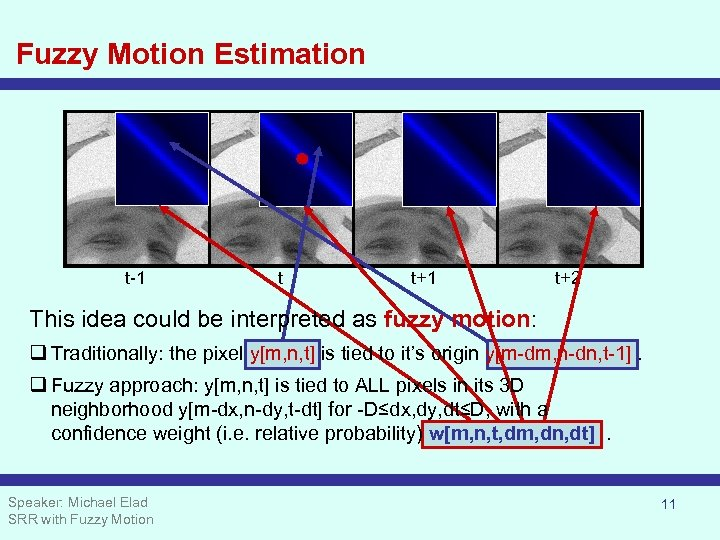Fuzzy Motion Estimation t-1 t t+1 t+2 This idea could be interpreted as fuzzy motion: q Traditionally: the pixel y[m, n, t] is tied to it’s origin y[m-dm, n-dn, t-1]. q Fuzzy approach: y[m, n, t] is tied to ALL pixels in its 3 D neighborhood y[m-dx, n-dy, t-dt] for -D≤dx, dy, dt≤D, with a confidence weight (i. e. relative probability) w[m, n, t, dm, dn, dt]. Speaker: Michael Elad SRR with Fuzzy Motion 11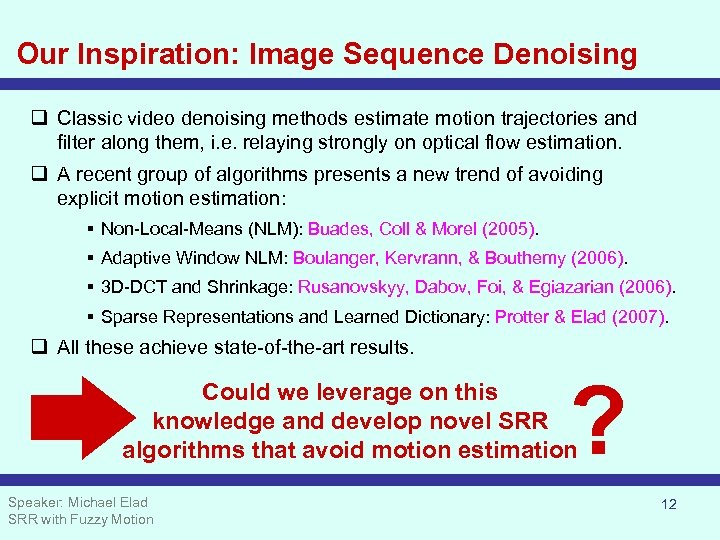Our Inspiration: Image Sequence Denoising q Classic video denoising methods estimate motion trajectories and filter along them, i. e. relaying strongly on optical flow estimation. q A recent group of algorithms presents a new trend of avoiding explicit motion estimation: § Non-Local-Means (NLM): Buades, Coll & Morel (2005). § Adaptive Window NLM: Boulanger, Kervrann, & Bouthemy (2006). § 3 D-DCT and Shrinkage: Rusanovskyy, Dabov, Foi, & Egiazarian (2006). § Sparse Representations and Learned Dictionary: Protter & Elad (2007). q All these achieve state-of-the-art results. ? Could we leverage on this knowledge and develop novel SRR algorithms that avoid motion estimation Speaker: Michael Elad SRR with Fuzzy Motion 12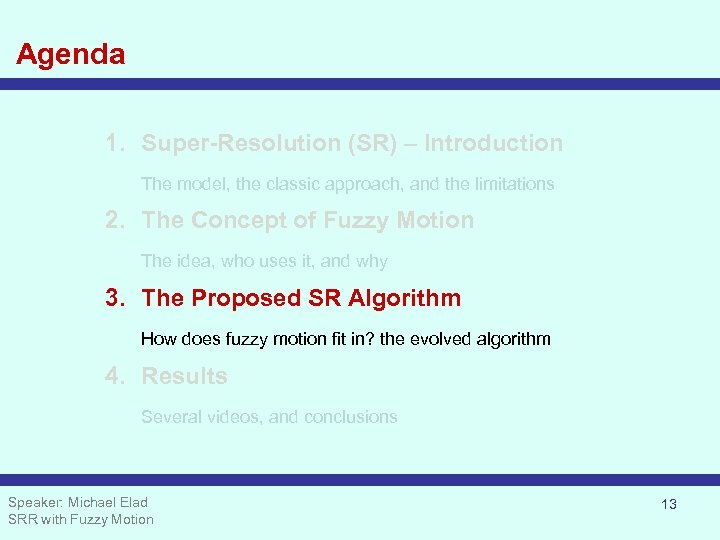Agenda 1. Super-Resolution (SR) – Introduction The model, the classic approach, and the limitations 2. The Concept of Fuzzy Motion The idea, who uses it, and why 3. The Proposed SR Algorithm How does fuzzy motion fit in? the evolved algorithm 4. Results Several videos, and conclusions Speaker: Michael Elad SRR with Fuzzy Motion 13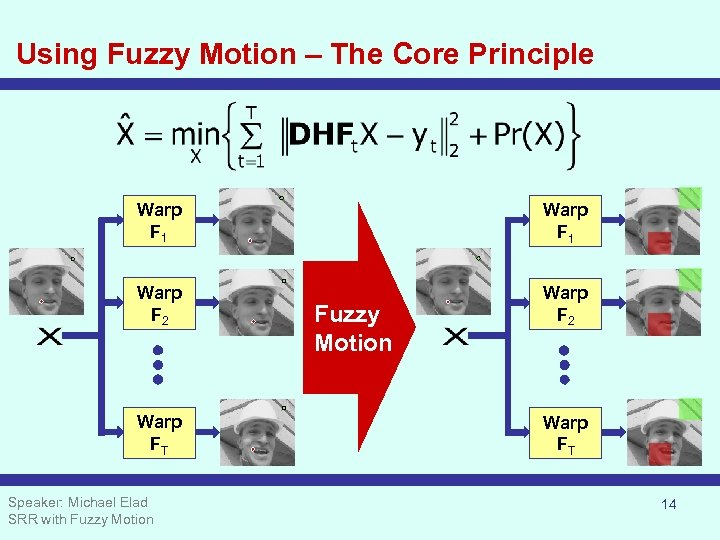Using Fuzzy Motion – The Core Principle Warp F 1 Warp F 2 Warp FT Speaker: Michael Elad SRR with Fuzzy Motion Warp FT 14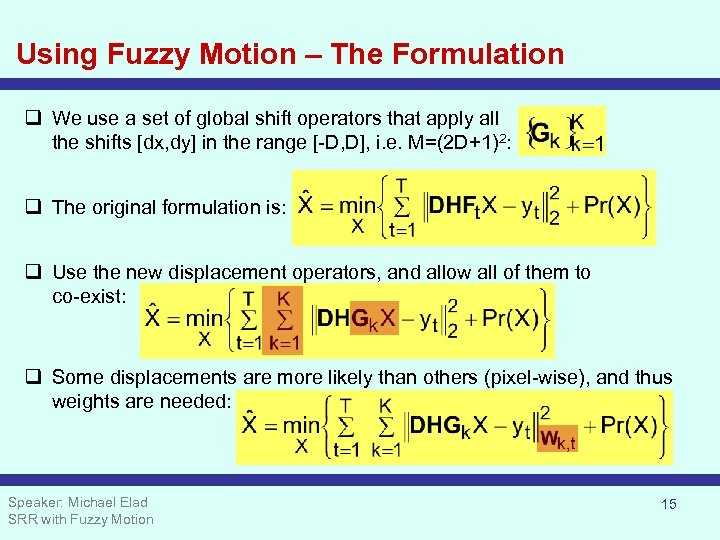Using Fuzzy Motion – The Formulation q We use a set of global shift operators that apply all the shifts [dx, dy] in the range [-D, D], i. e. M=(2 D+1)2: q The original formulation is: q Use the new displacement operators, and allow all of them to co-exist: q Some displacements are more likely than others (pixel-wise), and thus weights are needed: Speaker: Michael Elad SRR with Fuzzy Motion 15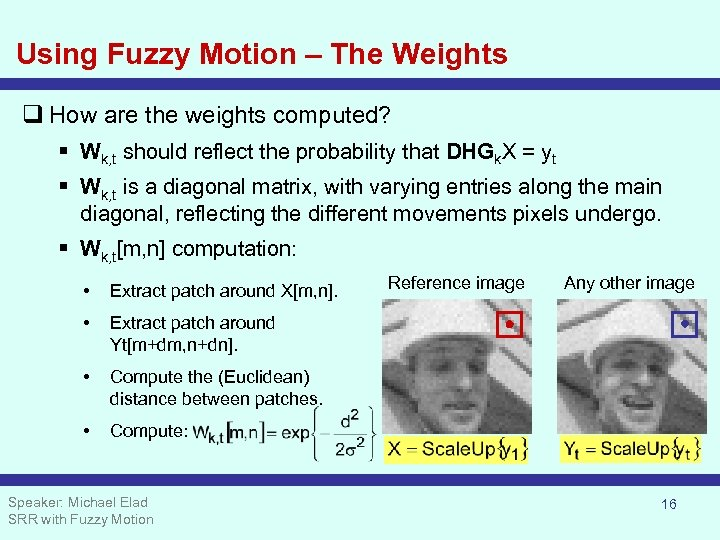Using Fuzzy Motion – The Weights q How are the weights computed? § Wk, t should reflect the probability that DHGk. X = yt § Wk, t is a diagonal matrix, with varying entries along the main diagonal, reflecting the different movements pixels undergo. § Wk, t[m, n] computation: • Extract patch around X[m, n]. • Compute the (Euclidean) distance between patches. • Any other image Extract patch around Yt[m+dm, n+dn]. • Reference image Compute: Speaker: Michael Elad SRR with Fuzzy Motion 16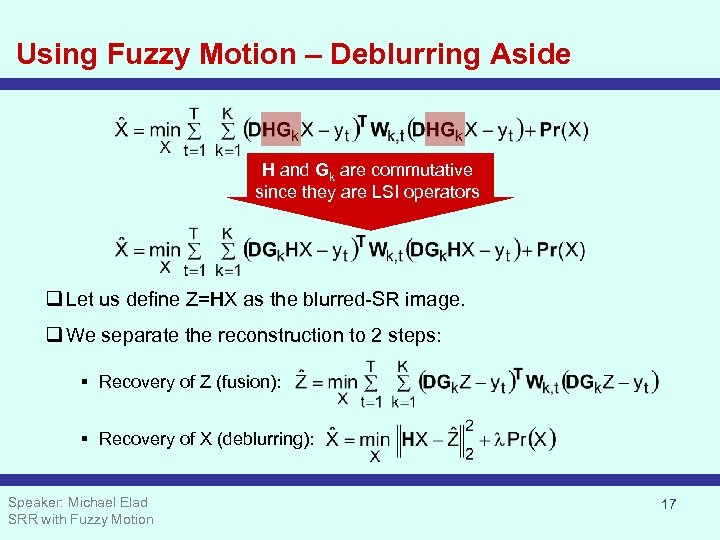Using Fuzzy Motion – Deblurring Aside H and Gk are commutative since they are LSI operators q Let us define Z=HX as the blurred-SR image. q We separate the reconstruction to 2 steps: § Recovery of Z (fusion): § Recovery of X (deblurring): Speaker: Michael Elad SRR with Fuzzy Motion 17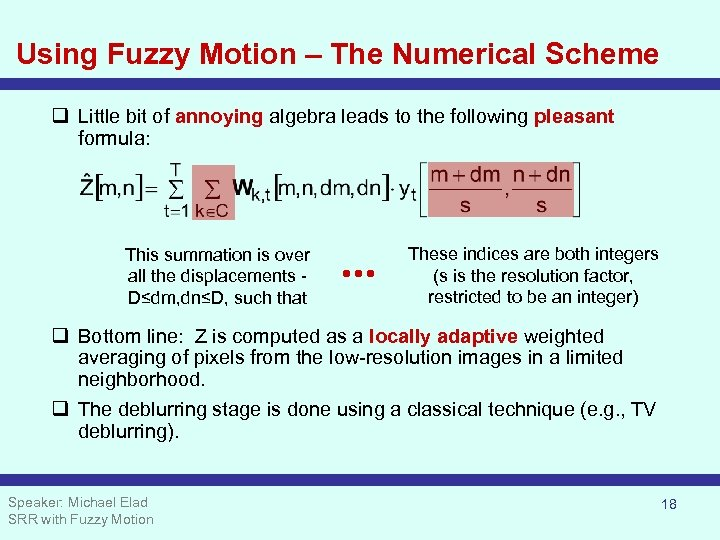Using Fuzzy Motion – The Numerical Scheme q Little bit of annoying algebra leads to the following pleasant formula: This summation is over all the displacements D≤dm, dn≤D, such that These indices are both integers (s is the resolution factor, restricted to be an integer) q Bottom line: Z is computed as a locally adaptive weighted averaging of pixels from the low-resolution images in a limited neighborhood. q The deblurring stage is done using a classical technique (e. g. , TV deblurring). Speaker: Michael Elad SRR with Fuzzy Motion 18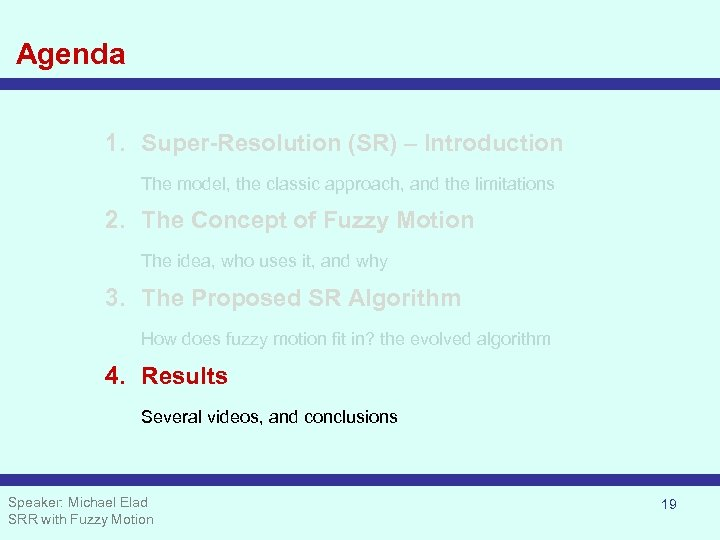Agenda 1. Super-Resolution (SR) – Introduction The model, the classic approach, and the limitations 2. The Concept of Fuzzy Motion The idea, who uses it, and why 3. The Proposed SR Algorithm How does fuzzy motion fit in? the evolved algorithm 4. Results Several videos, and conclusions Speaker: Michael Elad SRR with Fuzzy Motion 19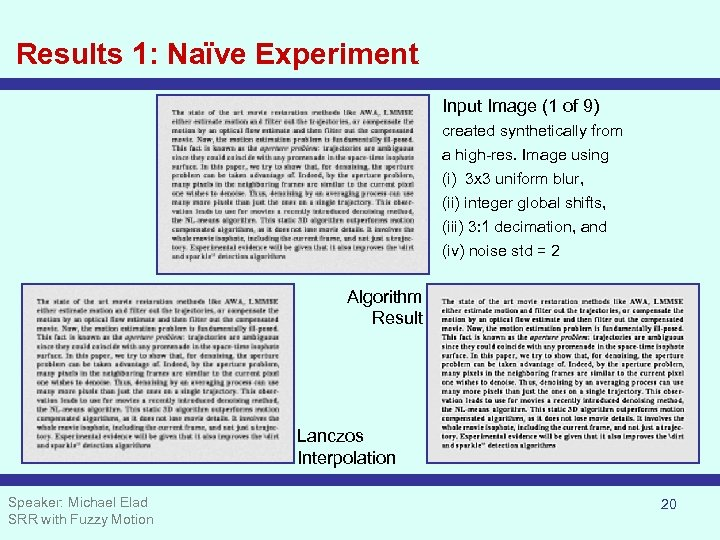Results 1: Naïve Experiment Input Image (1 of 9) created synthetically from a high-res. Image using (i) 3 x 3 uniform blur, (ii) integer global shifts, (iii) 3: 1 decimation, and (iv) noise std = 2 Algorithm Result Lanczos Interpolation Speaker: Michael Elad SRR with Fuzzy Motion 20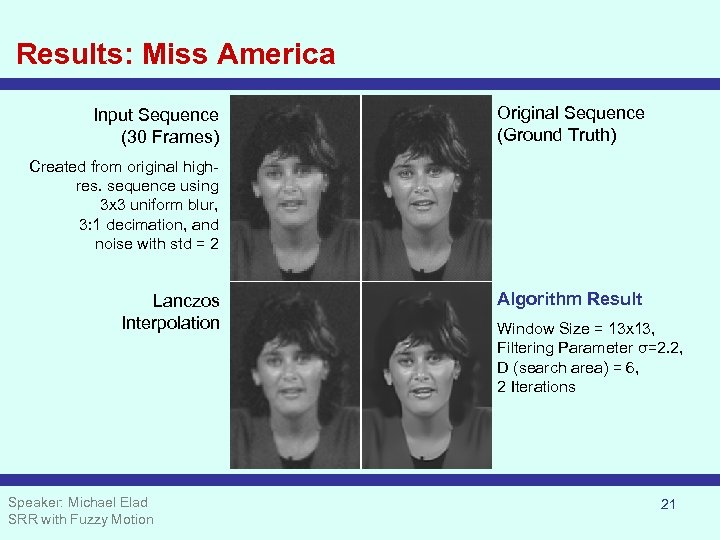Results: Miss America Input Sequence (30 Frames) Original Sequence (Ground Truth) Created from original highres. sequence using 3 x 3 uniform blur, 3: 1 decimation, and noise with std = 2 Lanczos Interpolation Speaker: Michael Elad SRR with Fuzzy Motion Algorithm Result Window Size = 13 x 13, Filtering Parameter σ=2. 2, D (search area) = 6, 2 Iterations 21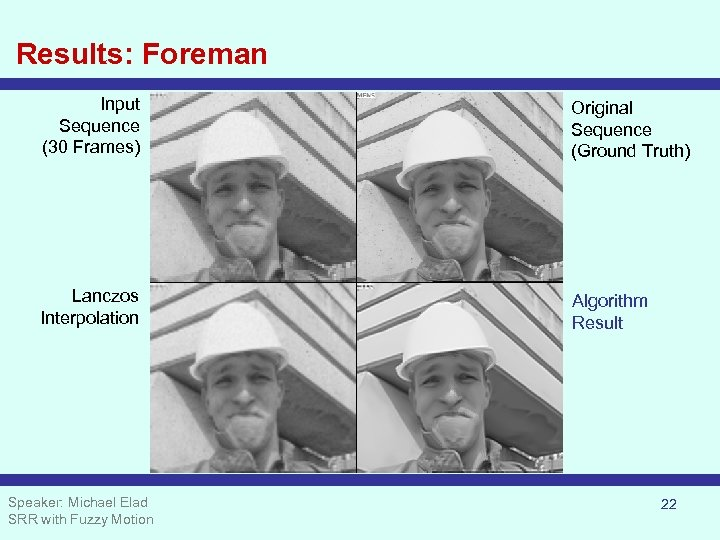Results: Foreman Input Sequence (30 Frames) Original Sequence (Ground Truth) Lanczos Interpolation Algorithm Result Speaker: Michael Elad SRR with Fuzzy Motion 22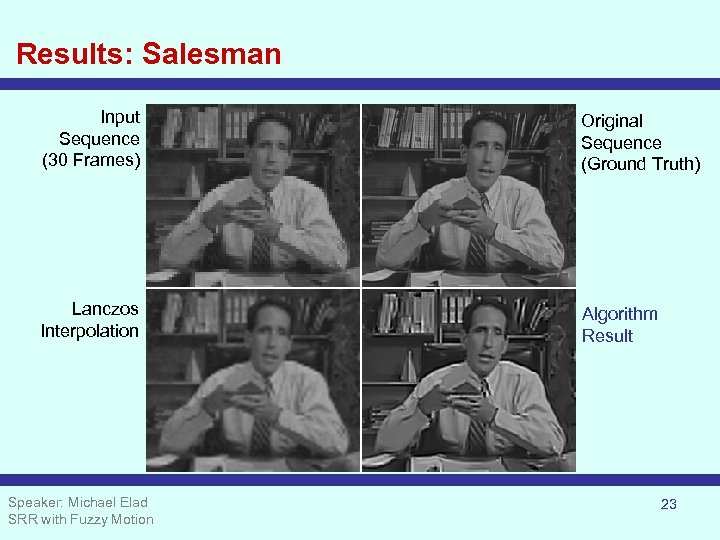Results: Salesman Input Sequence (30 Frames) Original Sequence (Ground Truth) Lanczos Interpolation Algorithm Result Speaker: Michael Elad SRR with Fuzzy Motion 23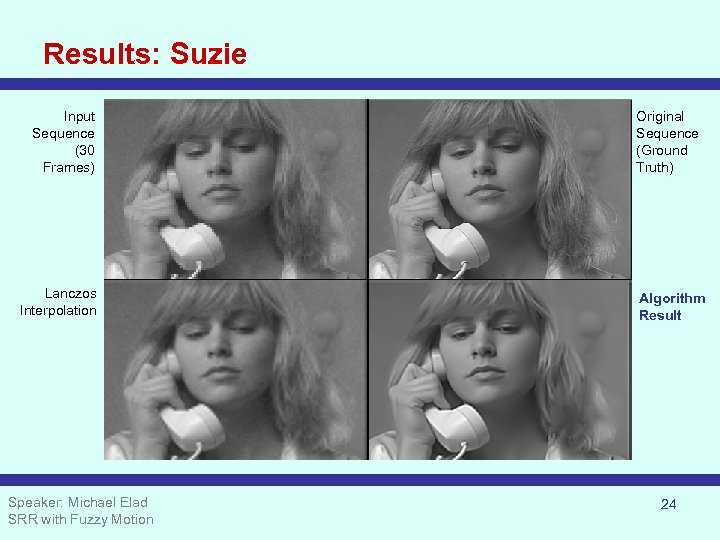Results: Suzie Input Sequence (30 Frames) Lanczos Interpolation Speaker: Michael Elad SRR with Fuzzy Motion Original Sequence (Ground Truth) Algorithm Result 24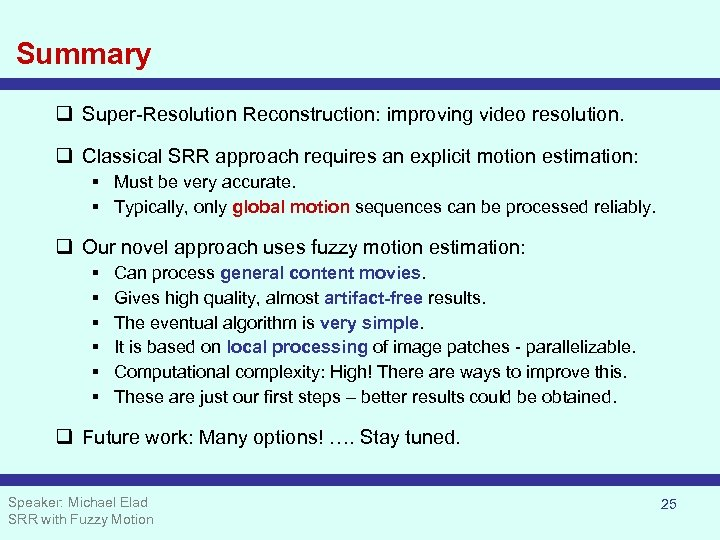Summary q Super-Resolution Reconstruction: improving video resolution. q Classical SRR approach requires an explicit motion estimation: § Must be very accurate. § Typically, only global motion sequences can be processed reliably. q Our novel approach uses fuzzy motion estimation: § § § Can process general content movies. Gives high quality, almost artifact-free results. The eventual algorithm is very simple. It is based on local processing of image patches - parallelizable. Computational complexity: High! There are ways to improve this. These are just our first steps – better results could be obtained. q Future work: Many options! …. Stay tuned. Speaker: Michael Elad SRR with Fuzzy Motion 25